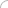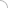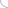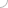# Area - aptitude test

Home > Aptitude test > Area

##### 1) When folded into two equal halves a rectangular sheet had a perimeter of 48cm for each part folded along one set of sides and the same is 66cm when folded along the other set of sides. Find the area of the sheet.

a) 1584
b) 1120
c) 792
d) 1320

SOLUTION :

Let the sheet be folded along its breadth and its perimeter = 48cm
Therefore, (l/2 + b) = 48 …... (i)
Now, let the sheet be folded along its length, and the perimeter = 66cm
(l + b/2)= 66 …... (ii)
Solving (i) and (ii), we get,
l = 56cm, b = 20cm
Area = l*b
Area = 1120 cm2

##### 2) Find the area of a rectangular field if John walking at the speed of 48m/min took 20 seconds to cross the field diagonally and Harry walking at a speed of 72m /min took 15 seconds to cross the field along its sides.

a) 64
b) 144
c) 34
d) Cannot be determined

SOLUTION :

The length of the diagonal = speed of John * time = (48/60)*20 = 16m
Sum of the adjacent sides of the field = speed of Harry * time = (72/60)*15 = 18m
Area of the field = (182 - 162) / 2 = 34m2

##### 3) If the length is increased by 25%, by what percent the width of a rectangle should be decreased so as to keep the area same.

a) 25%
b) 20%
c) 30%
d) 10%

SOLUTION :

Let the original length be l and the width be b
Therefore, the area = l*b
Now, as the length is increased by 25%, the new length is (1.25*l) and let the new width be x.
As the area is same, 1.25*l*x = l*b
x = b/1.25 = 0.8b
Therefore, the width is to be decreased by 20%.

##### 4) What is the length of the diagonal of a square whose area is 4 times of another square with diagonal as 5v2 cm?

a) 20v2
b) 10
c) 10v2
d) 20

SOLUTION : 10v2

Area of square = 1/2 * (length of diagonal)2
Area of square 1 = * (5v2)2 = 25
Area of square 2 = 4*25 = 100
Length of diagonal of square 2 = v2 * area = v2 * 100 = v200 = 10v2 cm

##### 5) The ratio of the areas of a square and rhombus whose base is same is:

a) 1:2
b) 2:1
c) 1:1
d) 3:1Aptitude questions